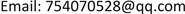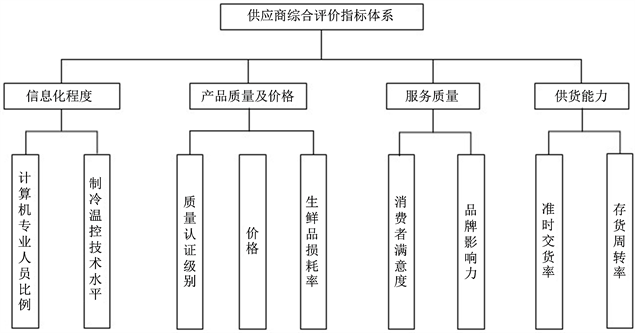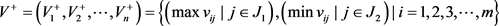﻿ 基于熵权的TOPSIS生鲜电商企业供应商选择研究 Study on Supplier Selection of Fresh E-Commerce Enterprises Based on Entropy Weight TOPSIS

Management Science and Engineering
Vol. 08  No. 01 ( 2019 ), Article ID: 28708 , 6 pages
10.12677/MSE.2019.81001

Study on Supplier Selection of Fresh E-Commerce Enterprises Based on Entropy Weight TOPSIS

Dan Liu

Beijing Jiaotong University, BeijingReceived: Jan. 8th, 2019; accepted: Jan. 22nd, 2019; published: Jan. 29th, 2019ABSTRACT

This article constructs the index system of supplier selection for fresh e-commerce companies from the four dimensions of informatization level, product quality and price, service quality and supply capacity. The entropy weight method was used to determine the weights of the evaluation indicators, and the TOPSIS method was used to comprehensively select suppliers of fresh e-commerce providers. Finally, an example was used for verification.

Keywords:TOPSIS, Entropy Value, Fresh Food Supplier, Supplier SelectionCopyright © 2019 by author(s) and Hans Publishers Inc.1. 引言

2. 生鲜电商企业供应商选择指标体系的建立

2.1. 供应商选择的评价指标Figure 1. Comprehensive evaluation index system for suppliers

2.2. 指标值的规范化(1)

${P}_{ij}=\left\{\frac{{x}_{ij}}{{\sum }_{i=1}^{m}\text{ }{x}_{ij}}\right\}$ (2)

2.3. 构建加权规范化矩阵

${e}_{j}=-k{\sum }_{i=1}^{m}{p}_{ij}\mathrm{ln}{p}_{ij}$ (3)

${w}_{j}=\frac{{h}_{j}}{{\sum }_{j=1}^{n}\text{ }{h}_{j}}$ (4)

3. TOPSIS方法的应用

TOPSIS是一种有效的多目标决策分析方法，又称逼近理想解排序法，借助多属性问题的理想解负理想解进行排序  。理想解是一个不存在的虚拟解，它的各项指标都符合供应商(评价对象)中的最好值；而负理想解是设想出来的最差解，它的各项指标都达到供应商(评价对象)的最坏解。

TOPSIS方法是通过规范化评价指标矩阵，找到矩阵中的理想解和负理想解。之后分别计算各供应商(评价对象)与理想值和负理想值的距离，将得到的距离进行排序，找到最接近理想解又最远离负理想解的评价对象。

Step 1 对权重矩阵 ${w}_{j}$ ，计算加权规范化指标矩阵V：

${V}_{ij}={W}_{j}{P}_{ij}=\left(\begin{array}{ccc}{w}_{1}{p}_{11}& \cdots & {w}_{n}{p}_{1n}\\ ⋮& \ddots & ⋮\\ {w}_{1}{p}_{m1}& \cdots & {w}_{n}{p}_{mn}\end{array}\right)$ (5)

Step 2 确定指标理想值和负理想值，分别构成理想值向量 ${V}^{+}$ ，负理想值 ${V}^{-}$(6)

${V}^{-}=\left({V}_{1}^{-},{V}_{2}^{-},\cdots ,{V}_{n}^{-}\right)=\left\{\left(\mathrm{min}{v}_{ij}|j\in {J}_{1}\right),\left(\mathrm{max}{v}_{ij}|j\in {J}_{2}\right)|i=1,2,3,\cdots ,m\right\}$ (7)

Step 3 计算各供应商(评价对象)的评价值与理想解和负理想解的距离

${D}_{i}^{+}=\sqrt{{\sum }_{j=1}^{n}{\left({V}_{ij}-{V}_{j}^{+}\right)}^{2}}$ (8)

${D}_{i}^{-}=\sqrt{{\sum }_{j=1}^{n}{\left({V}_{ij}-{V}_{j}^{-}\right)}^{2}}$ (9)

$i=1,2,3,\cdots ,m$

Step 4 计算各供应商(评价对象)的评价值向量与理想值向量之间的相对接近度：

${S}_{i}=\frac{{D}_{i}^{-}}{{D}_{i}^{+}+{D}_{i}^{-}}$ (10)

Step 5 按照接近度大小排序确定最优供应商(评价对象)：

${S}_{i}=\text{max}\left({S}_{1},{S}_{2},{S}_{3},\cdots ,{S}_{m}\right)$ (11)

4. 案例分析

$W=\left(0.9405215,0.0021741,0.0058970,0.0018325,0.0260415,0.0001331,0.0233463,0.0000442,0.0000096\right)$

5. 结论

Study on Supplier Selection of Fresh E-Commerce Enterprises Based on Entropy Weight TOPSIS[J]. 管理科学与工程, 2019, 08(01): 1-6. https://doi.org/10.12677/MSE.2019.81001

1. 1. 靖鲲鹏, 王佳岐. 基于因子分析的生鲜电商供应链评价指标体系研究[J]. 物流技术, 2018, 37(8): 103-107, 116.

2. 2. 艾瑞咨询. 中国生鲜电商行业消费洞察报告[R]. 艾瑞网, 2018.

3. 3. 季旭, 齐贺, 李飞, 欧海豪. 生鲜电商供应链研究[J]. 商场现代化, 2016(21): 32-33.

4. 4. 吴冲, 万翔宇. 基于改进熵权法的区间直觉模糊TOPSIS方法[J]. 运筹与管理, 2014, 23(5): 42-47.

5. 5. 雷静. 生鲜农产品电子商务供应链优化发展对策研究[J]. 天津经济, 2017(3): 21-24.

6. 6. 岳超源. 决策理论与方法[M]. 北京: 科学出版社, 2003.